Home | | Engineering Mathematics I | Functions of several Variables

# Functions of several Variables

· Problems based on Partial Derivatives · Problems based on Euler`s Theorem · Problems based on Total Derivatives-Differentiation of Implicit Function · Problems based on Jacobian · Problems based on Taylor`s and Laurent Series · Problems based on Maxima and Minima for Functions of Two Variables · Problems based on Lagrangian Multiplier

Functions of several Variables

· Problems based on Partial Derivatives

· Problems based on Euler`s Theorem

· Problems based on Total Derivatives-Differentiation of Implicit Function

· Problems based on Jacobian

· Problems based on Taylor`s and Laurent Series

· Problems based on Maxima and Minima for Functions of Two Variables

· Problems based on Lagrangian Multiplier

Partial Derivatives

Partial Derivatives: Let z=f(x,y) be a function of two Variables x and y, If we keep y as a constant and Vary x alone , then z is a function of x only ,

The derivative of z w.r.to x, treating y as a constant is called the partial derivatives w.r.to x and it is denoted by the symbolsSuccessive Partial Differentiation: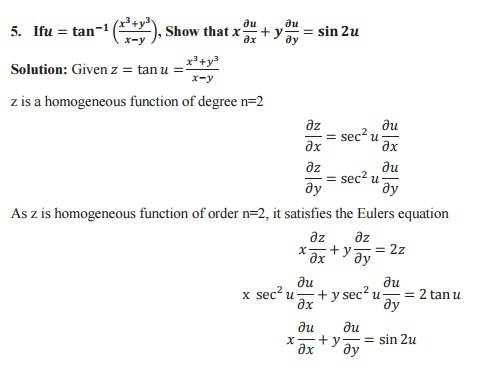Euler`s Theorem for Homogeneous Function

Euler`s Theorem: If u be a homogeneous function of degree n an x and y thenTotal Derivatives-Differentiation of Implicit Function

Total Derivative: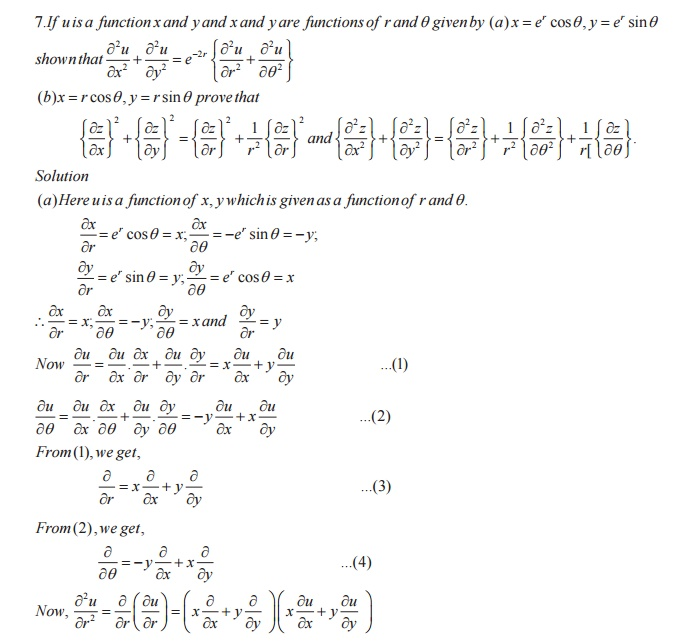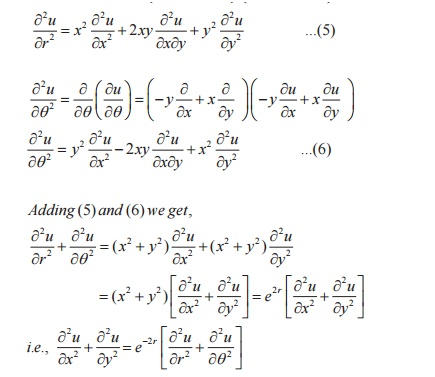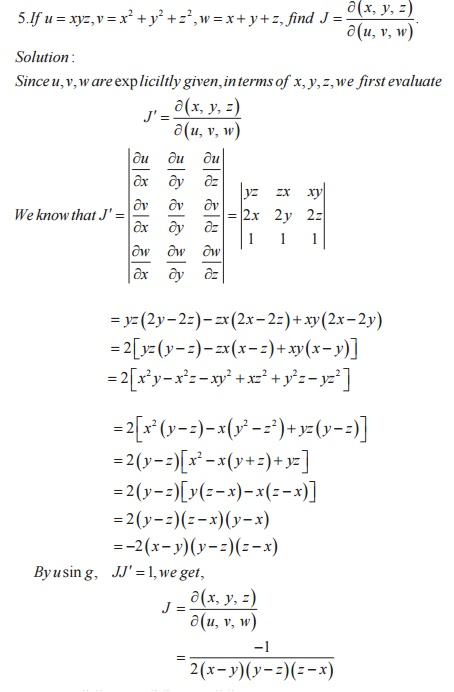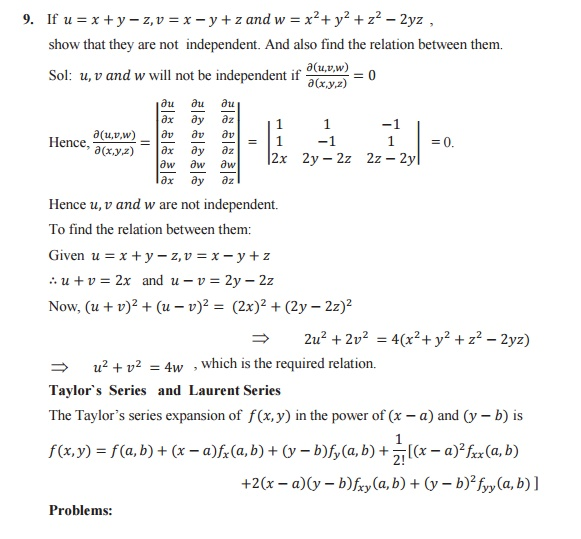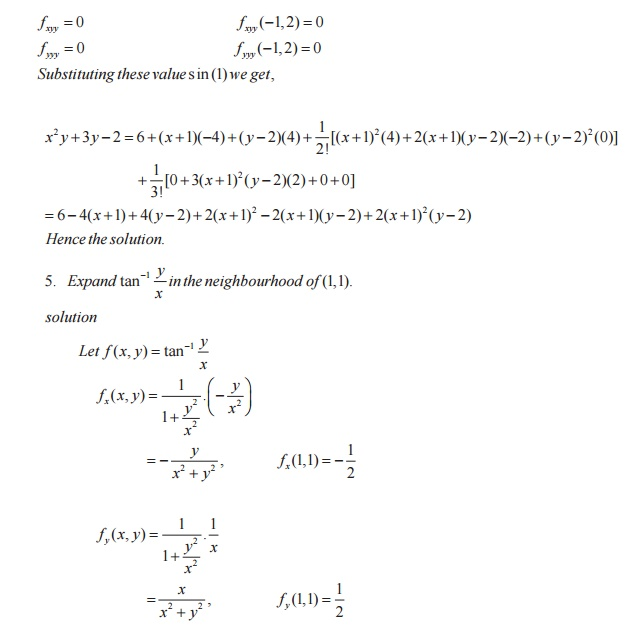Maxima and Minima and Lagrangian Multiplier

Defn: Maximum Value

if f(a,b) is a maximum value of (x,y) if their exists some neighbourhood of the point (a,b) such that for every point (a+h,b+k) of the neighbourhood

f(a,b)>f(a+h,b+k)

Defn: Minimum Value

if f(a,b) is a maximum value of (x,y) if their exists some neighbourhood of the point (a,b) such that for every point (a+h,b+k) of the neighbourhood

f(a,b)<f(a+h,b+k)

Defn: Extremum Value

if f(a,b) is said to be an extremum value o if f(a,b) it is maximum or minimum

Defn: Lagrangian Multiplier

Suppose we require to find the maximum and minimum values of (x,y,z)  where x,y,z are subject to a constraint equation g(x,y,z)=0

We define a function F(x,y,z) = f(x,y,z) + λg(x,y,z) where λ is called Lagrangian Multiplier which is independent of x,y,z.

Problems: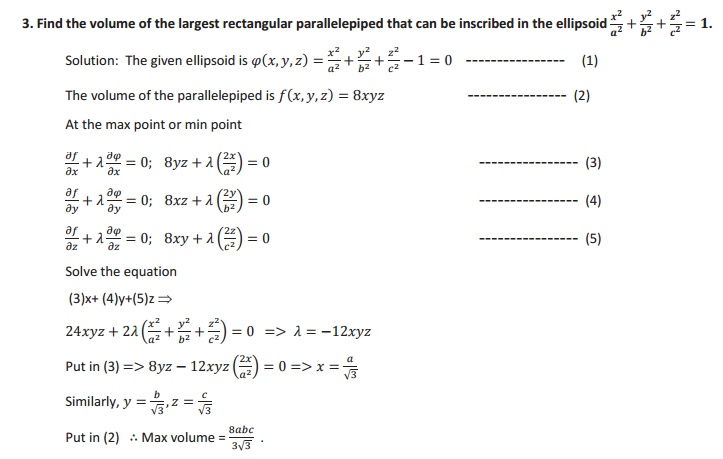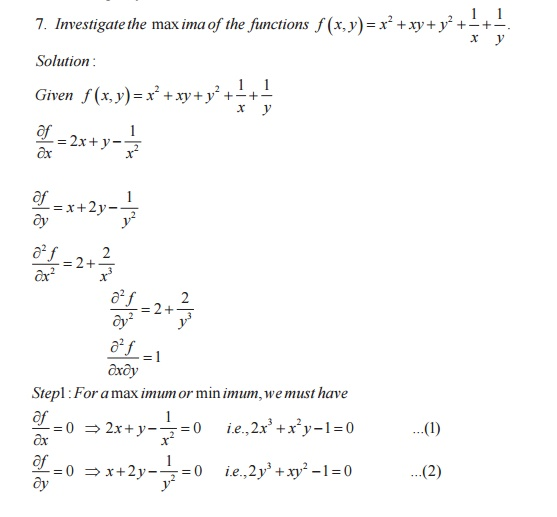Study Material, Lecturing Notes, Assignment, Reference, Wiki description explanation, brief detail
Mathematics : Functions of several Variables : Functions of several Variables |

Related Topics